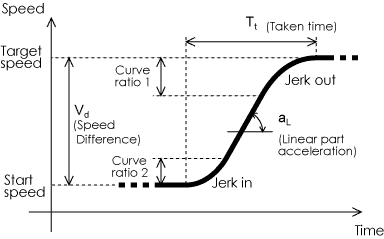# SJ300-EL Parameter Calculating Program for Constant Jerk Rate

 Acceleration pattern selection Elevator S curve Deceleration pattern selection Elevator S curve Max frequency Speed at Max frequency Frequency Conversion Function Enable Speed unit of Max freqency(A075) *note m/s (or other L/T) feet/minJerk in Jerk out Max aL Curve ratio 1 Curve ratio 2 During Acc During Dec

Meaning of remarks
 L The speed difference is smaller than one tenth of max speed. Therefore, the acceleration or deceleration will be linear. D It is not possible to decelerate from this multi-speed to 7th multi speed (creep) with the specified jerk rate. To decelerate to creep speed from this multi-speed with specified jerk rate, define one more creep speed for deceleration from this multi-speed. Acceleration time is calculated for acceleration from 0 Hz to yield specified jerk rate.

For acceleration or deceleration to any multi-speed, the acceleration or deceleration time of the target multi-speed will be used except for athe 7th multi-speed. 7th multi-speed is used for creep speed. When decelerating from other other (1-6) to this 7th speed, the deceleration time of the prevous multi-speed will be used. Therefore deceleration time of each speed is calculated for the deceleration to creep speed with specified jerk rate, except the case when the speed is decelerated from multi-speed 1-6.

When decelerating from multi-speed 1-6 speed to another multi-speed, the deceleration time is calculated for the deceleration to yield the specified jerk rate and the speed will show remark "D". This case is considered to be rare for the normal elevator application and it is not possible to decelerate from this multi-speed to 7th (creep) speed with the specified jerk rate. However the specified jerk rate for deceleration can be accomplished by defining one more creep speed for the deceleration from this multi speed (The defined creep speed will show remark "D").

In this program 0 Hz is assigned for multi-speed 0, though it is not always necessary in general use. This deceleration time (F003) is calculated base on decelerating to this speed (0 Hz) from 7th (creep) speed with specified jerk rate.

F002 is not used and fixed to factory default value.

F003 is calculated for a deceleration from multi-speed 7 (creep speed) to yield specified jerk rate. However this deceleration may be linear, if creep speed is less than one tenth of Max frequency.

A227 is calculated for acceleration from 0 Hz to yield specified jerk rate.

A327 is calculated for deceleration to 0 Hz to yield specified jerk rate.